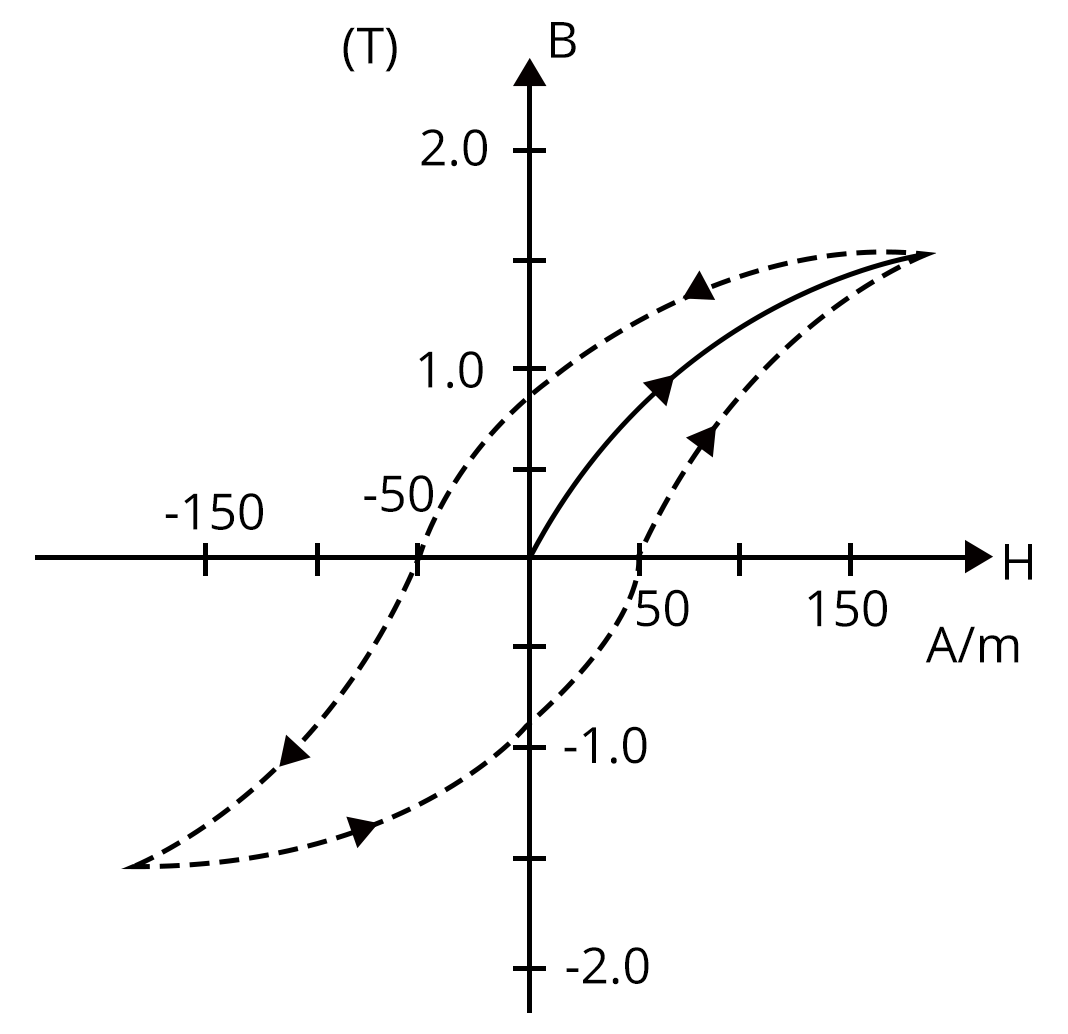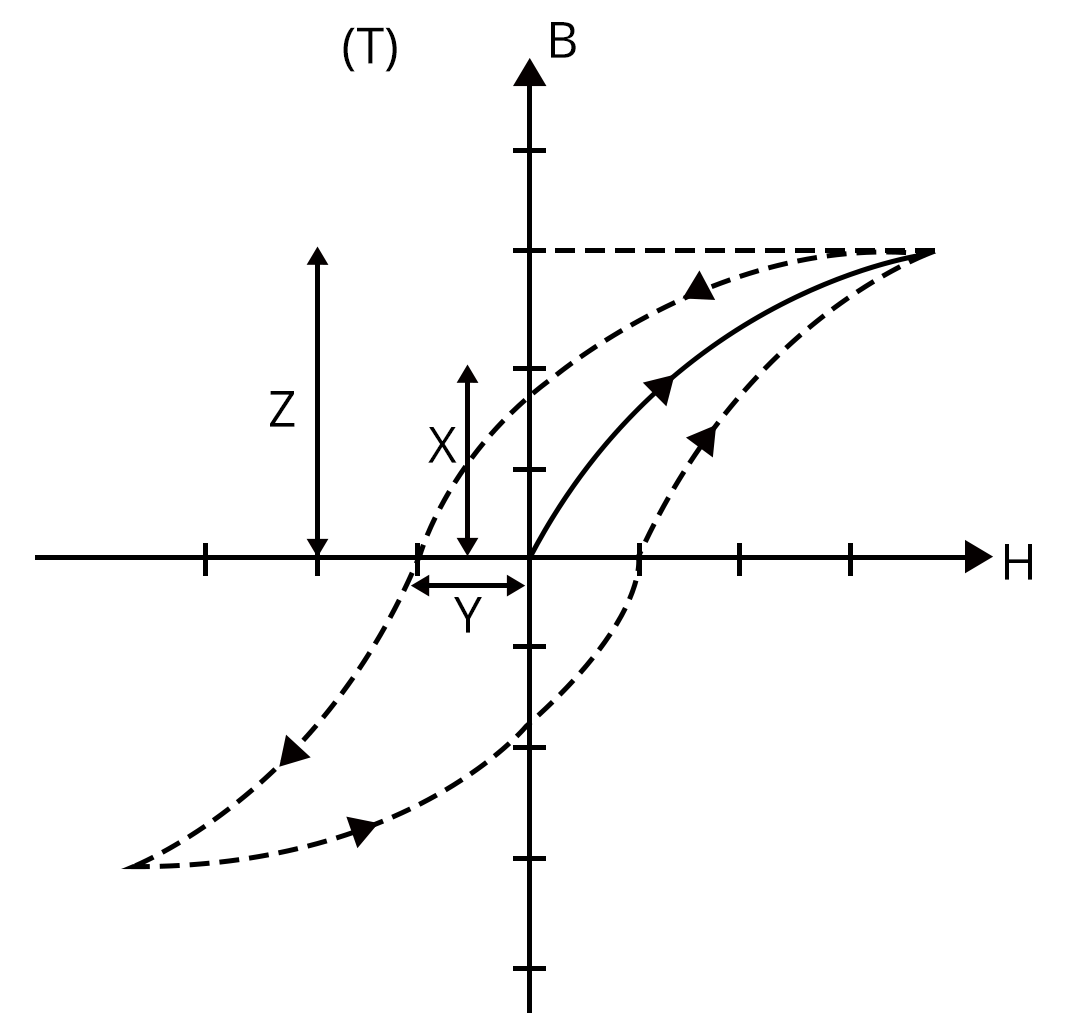Courses
Courses for Kids
Free study material
Offline Centres
More

# Magnetic Effects of Current and Magnetism Chapter - Physics JEE MainLast updated date: 01st Dec 2023
Total views: 21k
Views today: 0.21k## Concepts of Magnetic Effects of Current and Magnetism for JEE Main Physics

The chapter magnetic effects of electric current deals with the magnetic field produced by the current-carrying wire and moving charges and also magnetic force acting on a current-carrying wire. In magnetism, properties of a magnetic dipole, earth’s magnetism and different magnetic material are discussed. From JEE's point of view, students can score good marks in these chapters with proper practice and effort.

In magnetic effects of current, problems based on moving charges in a magnetic field and their applications are important. The magnetic field of various current-carrying conductors of various shapes and solenoids are derived using Biot Savart law and Ampere’s law. The force acting on a current-carrying conductor in a magnetic field is also important. Here, we will see many magnetic effects of electric current examples.

In the section on magnetism, earth’s magnetism and magnetic materials are very important and problems based on a magnetic bar placed in an external magnetic field are also discussed in detail. Also, new terms related to the magnetisation of material are also discussed along with the hysteresis curve.

Now, let us move on to the important concepts and formulae related to JEE and JEE Main exams from magnetic effects of current  and magnetism along with a few solved magnetic effects of electric current examples.

## JEE Main Physics Chapters 2024

 JEE Main Physics Chapters 1 Units and Measurement 11 Electrostatics 2 Kinematics 12 Current Electricity 3 Laws of Motion 13 Magnetic Effects of Current and Magnetism 4 Work, Energy and Power 14 Electromagnetic Induction and Alternating Currents 5 Rotational Motion 15 Electromagnetic Waves 6 Gravitation 16 Optics 7 Properties of Solids and Liquids 17 Dual Nature of Matter 8 Thermodynamics 18 Atoms and Nuclei 9 Kinetic Theory of Gases 19 Electronic Devices 10 Oscillations and Waves 20 Communication Systems

### Important Topics of Magnetic Effects of Current and Magnetism Chapter

• Magnetic force on a moving charge in a magnetic field

• Trajectory of a charge moving in a perpendicular magnetic field

• Cyclotron

• Biot Savart Law

• Ampere’s Law

• Magnetic field due to a circular and straight wire

• Magnetic field due to a solenoid

• Force on a current-carrying conductor in a magnetic field.

• The force between two parallel current-carrying conductors

• Magnetic dipole moment of a magnet

• Terms related to magnetism

• Elements of Earth’s Magnetic Field

• Magnetic Materials

• Curie’s Law

• Hysteresis curve

### JEE Main Magnetic Effects of Current and Magnetism Solved Examples

1. A current of 10 A is flowing in a wire of a length of 1.5 m. A force of 15 N acts on it when it is placed in a uniform magnetic field of 2 T. The angle between the direction of the current and magnetic field is…

Sol:

Given,

Uniform magnetic field, B = 2T

Current passing through the wire, I = 10 A

Length of wire, L = 1.5 m

The force acting on the wire placed in the magnetic field, F = 15 N

The formula to calculate the magnetic force acting on the wire is given by,

$F=ILB\sin\theta$

Substitute values for each term in the formula to calculate the angle between the magnetic field and direction of the current.

$15=10\times 1.5\times 2\sin\theta$

$\sin\theta=\dfrac{1}{2}$

$\theta=\sin^{-1}(0.5)$

$\theta=30^{0}$

Therefore, the angle between the direction of the current and magnetic field is 30o.

Key Point: A current-carrying placed in a magnetic field experience a force.

1. If the angle of dip at two places are 300 and 450 respectively, then the ratio of horizontal components of earth’s magnetic field at two places will be

Sol:

Given,

The angle of dip of two places are θ1= 300 and  θ2=450

The horizontal component of earth’s magnetic field where θ1= 300 is given by,

$B_{H1}=B\cos\theta_1$

$B_{H1}=B\cos(30^0)$

$B_{H1}=\dfrac{\sqrt{3}B}{2}$...(1)

Similarly, the horizontal component of earth’s magnetic field where θ1= 450 is given by,

$B_{H2}=B\cos\theta_2$

$B_{H2}=B\cos(45^0)$

$B_{H2}=\dfrac{B}{\sqrt{2}}$....(2)

Divide equation (1) by equation (2) to obtain the ratio of horizontal component of earth’s magnetic field.

$\dfrac{B_{H1}}{B_{H2}}=\dfrac{\left(\dfrac{\sqrt{3}B}{2}\right)}{\left(\dfrac{B}{\sqrt{2}}\right)}$

$\dfrac{B_{H1}}{B_{H2}}=\dfrac{\sqrt{3}}{\sqrt{2}}$

Therefore, the ratio of horizontal components of earth’s magnetic field at two places is $\dfrac{\sqrt{3}}{\sqrt{2}}$.

Key point: The horizontal component of earth’s magnetic field and dip angle is related by the formula.

### Previous Year Questions from JEE paper

1. A proton, a deuteron and an α particle are moving with the same momentum in a uniform magnetic field. The ratio of magnetic forces acting on them is _______ and their speeds are in the ratio______.(JEE 2021)

a. 2 : 1 : 1 and 4 : 2 : 1

b. 1 : 2 : 4 and 2 : 1 :1

c. 1 : 2 : 4 and 1 : 1 : 2

d. 4 : 2 : 1 and 2 : 1 : 1

Sol:

We know velocity(v) of a body is related to its momentum by the formula

$v=\dfrac{P}{m}$

The magnetic force acting on a charged particle is given by the formula,

Let the mass of a proton, duetron and  α particle be m, 2m and 4m respectively.

Let the charge of a proton, duetron and  α particle be q, 2q and 4q respectively.

Force acting on proton in a magnetic field B is given by,

$F_1=qvB$

$F_1=q\left(\dfrac{P}{m}\right)B$

Force acting on duetron in a magnetic field B is given by,

$F_2=qvB$

$F_2=q\left(\dfrac{P}{2m}\right)B$

Force acting on α particle in a magnetic field B is given by,

$F_3=2qvB$

$F_3=2q\left(\dfrac{P}{4m}\right)B$

$F_3=q\left(\dfrac{P}{2m}\right)B$

The ratio of magnetic forces on the charged particles are,

$F_1:F_2:F_3=q\left(\dfrac{P}{m}\right)B:q\left(\dfrac{P}{2m}\right)B:q\left(\dfrac{P}{2m}\right)B$

$F_1:F_2:F_3=2:1:1$

The ratio of velocities of charge particles are,

$v_1:v_2:v_3=\left(\dfrac{P}{m}\right):\left(\dfrac{P}{2m}\right):\left(\dfrac{P}{4m}\right)$

$v_1:v_2:v_3=4:2:1$

Therefore, correct option is option (a)

Key Point: Knowing the charge and mass of proton, duetron and alpha particle is important in order to solve the above problem.

1. The figure gives experimentally measured B vs H variation in a ferromagnetic material. The retentivity, coercivity and saturation, respectively, of the material, are(JEE Main 2021)a. 1.5 T, 50 A/m, 1 T

b. 1 T, 50 A/m, 1.5 T

c. 1.5 T, 50 A/m, 1 T

d. 150 A/m, 1 T, 1.5 T

Sol:Retentivity is the ability of an object to retain magnetism even after the magnetising field is removed. It is denoted by the value of X = 1T

Coercivity is the opposing magnetising field required to demagnetise the material and it is denoted by the value of Y = 50 A/m

Saturation is the value of magnetic field density in the B-H curve denoted by the value of Z = 1.5 T

### Practice Questions

1. A magnet is parallel to a uniform magnetic field. If it is rotated by 60o, the work done is 0.8 J. How much work is done in moving 30o further? (Ans: 0.8 J)

2. A charged particle (charge q) is moving in a circle of radius R with a uniform speed v. The associated magnetic moment is given by μ (Ans: qvR/2)

## JEE Main Physics Magnetic Effects of Current and Magnetism Study Materials

Here, you'll find a comprehensive collection of study resources for Magnetic Effects of Current and Magnetism designed to help you excel in your JEE Main preparation. These materials cover various topics, providing you with a range of valuable content to support your studies. Simply click on the links below to access the study materials of Magnetic Effects of Current and Magnetism and enhance your preparation for this challenging exam.

 JEE Main Magnetic Effects of Current and Magnetism Study Materials JEE Main Magnetic Effects of Current and Magnetism Notes JEE Main Magnetic Effects of Current and Magnetism Important Questions JEE Main Magnetic Effects of Current and Magnetism Practice Paper

## JEE Main Physics Study and Practice Materials

Explore an array of resources in the JEE Main Physics Study and Practice Materials section. Our practice materials offer a wide variety of questions, comprehensive solutions, and a realistic test experience to elevate your preparation for the JEE Main exam. These tools are indispensable for self-assessment, boosting confidence, and refining problem-solving abilities, guaranteeing your readiness for the test. Explore the links below to enrich your Physics preparation.

 JEE Main Physics Study and Practice Materials JEE  Main Physics Previous Year Question Papers JEE Main Physics Mock Test JEE Main Physics Formula JEE Main Sample Paper JEE Main Physics Difference Between

### Conclusion

In this article, we'll delve into Magnetic Effects of Current and Magnetism" in your JEE Main physics chapter. You'll explore the core ideas, problem-solving approaches, and crucial concepts related to this topic. Learn about the intriguing forces and behaviors that electricity and magnetism exhibit. We've compiled all the essential information you need in one convenient place. You can easily access downloadable PDFs, providing detailed explanations and solutions to help you master this topic. These resources will be a valuable asset for your exam preparation, ensuring you're well-prepared to tackle any questions that come your way.

## FAQs on Magnetic Effects of Current and Magnetism Chapter - Physics JEE Main

1. What is the weightage of the laws of motion in JEE?

Nearly 1-2 questions are asked in the exam from this chapter covering about 10 marks.

2. What is the difficulty level of the chapter on magnetic effects of current and magnetism?

Students can easily answer the questions coming from the magnetism section if they have gone through the concepts thoroughly. However, the questions from magnetic effects of current requires knowledge and problem solving skills to score good marks.

3. Are previous year's questions Important for JEE Main?

Yes, previous year's JEE Main questions are important. Once the student is finished with learning concepts and solving problems, they must go through the previous year's question papers of the last 20 years to understand the test pattern and frequent questions asked in the exam.

### Repeaters Course for JEE 2022 - 23## Notice board

JEE News
JEE Blogs